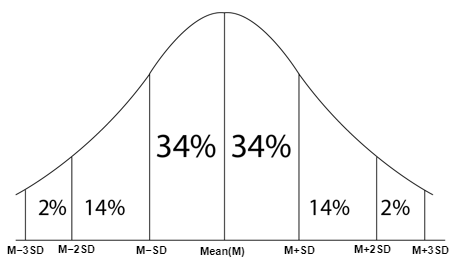# GRE Quantitative: Data Distributions GRE Practice Questions with solutions

The biggest question to bother your mind is why is Data Interpretation even included in the syllabus for GRE? Why should one consider data interpretation as an important topic?

All these answers lie in a single statement. There’s a famous proverb, “a picture is worth a million words,” and same applies to graphical and tabular representation. A single picture can give a million bits of information and is the best way of expressing business data in the least amount of space. No wonder so many grads and B-schools give such high importance to data interpretation.

Topics you need to pay attention to in data interpretation are listed below :

Data interpretation includes different topics and it is important for a GRE exam aspirant to be familiar with each topic. These topics are:

• Mean, Mode, Median, Range, Standard Deviation, Percentiles and Quartiles
• Bar charts, Pie charts, Line graphs, Histograms
• Best fit lines, Scatterplots, Boxplots
• Frequency Distributions
• Probability, Conditional Probability
• Random Variables, Probability Distributions, Normal Distributions
• Permutations and Combinations, Venn Diagram

Normal Distributions

Normal Distributions are bell-curved graphs depicting the distribution of data. This is called a normal distribution because most of the data is concentrated in the center of the data(Mean). The deviations are equal through both sides of the mean, and they usually do not contain extreme values.The midpoint of the graph is known as the mean.

The mean is depicted as m, and the deviation is depicted as d. When we say m+d and m-d, we mean that it is the first deviation after and before the mean. 34% of the data falls in between the two points on either side of the mean. m+ 2d and m-2d are the second deviations. Between the first and the second deviations, a total of 14% of the data falls either side of the mean.

Simplifying it, a total 68% of the data falls within the first deviations (both sides of the mean) and a total of approximately 96% of the data falls between both the second deviations(68 + 14 + 14).

The remaining 4% of the data falls between the second and the third deviations

(m + 3d , m – 3d) of the data.

Let’s see some data interpretation GRE questions with solutions and understand DI completely.Question: The total value is $340 million. On the basis of the pie chart given above, answer the following questions: 1. Using an approximate value, find the ratio of the value of sensitized products to the value of still-pictures produced? 2. If the value of office copiers produced was 30 percent greater than the corresponding value given, what would be the value of office copiers produced? 3. Find in an approximation, the ratio of microfilm products to motion pictures produced. Solution: 1. The ratio of the value of sensitized products to the value of still-picture products is equal to the ratio of their corresponding percentages as the denominator of percentage is always the same value, that is, the total value. So their ratio will be 49:10 or approximately 5:1. 2. The value of office copiers produced is 0.25 times$85 million, or $85 million. Therefore, if the corresponding value becomes x million dollars, then 1.3x = 85 million dollars. Solving for x gives, x= 85/1.3=65.39. Hence it is approximately$65.39.
3. Ratio of microfilm production to motion pictures produced = 8:3
Hence, it is approximately in a ratio of 3:1.

BYJU’S will be glad to help you in your GRE preparation journey. You can ask for any assistance related to GRE from us by just giving a missed call at +918884544444, or you can drop an SMS. You can write to us at gre@byjus.com.